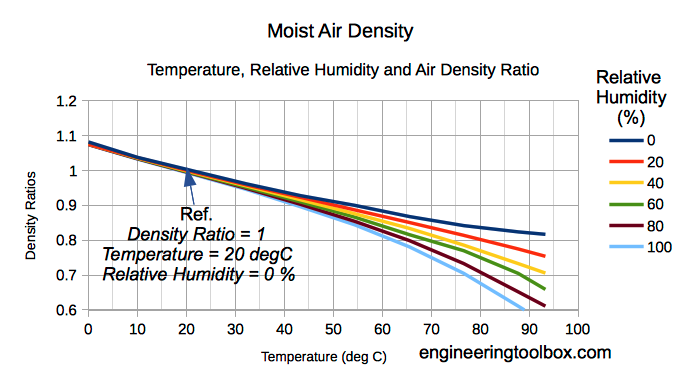Engineering ToolBox - Resources, Tools and Basic Information for Engineering and Design of Technical Applications!

# Moist Air - Density vs. Water Content and Temperature

## Density of the mix of dry air and water vapor - moist humid air.

The density of humid air varies with water content and temperature. When the temperature increases a higher molecular motion results in expansion of volume and a decrease of density.

The density of a gas, dry air, water vapor - or a mixture of dry air and water vapor like moist or humid air - can be calculated with the Ideal Gas Law.

### Density of Dry Air

The density of dry air can be calculated

ρa = pa / Ra T

= 0.0035 pa / T                                   (1)

where

ρa = density dry air (kg/m3)

pa = partial pressure of dry air (Pa, N/m2)

Ra = 286.9 - individual gas constant of dry air (J/kg K)

T = absolute dry bulb temperature (K)

### Density of Water Vapor

The density of the water vapor can be calculated

ρw = pw / Rw T

= 0.0022 pw / T                                 (2)

where

pw = partial pressure of water vapor (Pa, N/m2)

ρw = density of water vapor (kg/m3)

Rw = 461.5 - individual gas constant water vapor (J/kg K)

T = absolute dry bulb temperature (K)

### Density of Moist Air - an Air Vapor Mixture

The amount of water vapor in air influences the density. Water vapor is relatively light compared to diatomic Oxygen and diatomic Nitrogen - the dominant components in air.

When vapor content increases in moist air the amount of Oxygen and Nitrogen are decreased per unit volume and the density of the mix decreases since the mass is decreasing.

Based on specific volume of moist air the moist air density can be calculated

ρ = 1 / v

= (p / Ra T) (1 + x) / (1 + x Rw / Ra)                                          (3)

where

v = specific volume of moist air per mass unit of dry air and water vapor (m3/kg)

x = mw / ma = specific humidity or humidity ratio (kgh2o/kgdry_air)

mw = mass of water vapor (kg)

ma = mass of dry air (kg)

p = pressure in the moist air (Pa)

The density of dry air with the same total pressure p as in the mixture (dry air + vapor partial pressure) - can be calculated as a reference as

ρref = p / Ra T         (4)

where

ρref = reference density of dry air with the same pressure as in the air vapor mixture (kg/m3)

The density of moist air related to dry air can be expressed by combining (4) and (3) as

ρ = ρref (1 + x) / (1 + x Rw / Ra)                                       (5)

The gas constant ratio between water vapor and air is

Rw / Ra = (461.5 J/kg K) / (286.9 J/kg K)

= 1.609

and inserting the ratio in (5) the density of moist air related to dry air can be expressed as

ρ = ρref (1 + x) / (1 + 1.609 x )                                         (6)

Note! As we can see from (6) - increased moisture content reduces the density of moist air - dry air is more dense than moist air.## Related Topics

• Air Psychrometrics - Moist and humid air - psychrometric charts, Mollier diagrams, air-condition temperatures and absolute and relative humidity and moisture content.
• Gases and Compressed Air - Air, LNG, LPG and other common gas properties, pipeline capacities, sizing of relief valves.
• Densities - Densities of solids, liquids and gases. Definitions and convertion calculators.

## Engineering ToolBox - SketchUp Extension - Online 3D modeling!

Add standard and customized parametric components - like flange beams, lumbers, piping, stairs and more - to your Sketchup model with the Engineering ToolBox - SketchUp Extension - enabled for use with the amazing, fun and free SketchUp Make and SketchUp Pro .Add the Engineering ToolBox extension to your SketchUp from the SketchUp Pro Sketchup Extension Warehouse!

Translate

## Privacy

We don't collect information from our users. Only emails and answers are saved in our archive. Cookies are only used in the browser to improve user experience.

Some of our calculators and applications let you save application data to your local computer. These applications will - due to browser restrictions - send data between your browser and our server. We don't save this data.

## Citation

• Engineering ToolBox, (2004). Moist Air - Density vs. Water Content and Temperature. [online] Available at: https://www.engineeringtoolbox.com/density-air-d_680.html [Accessed Day Mo. Year].

Modify access date.

. .

#### Scientific Online Calculator3 30